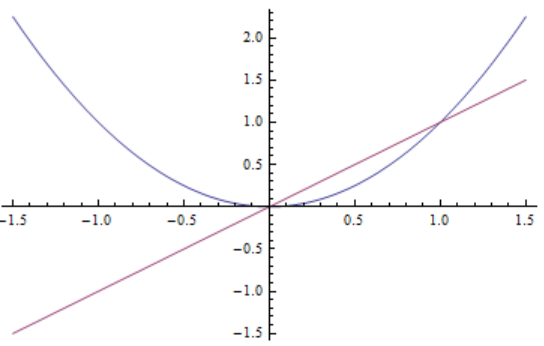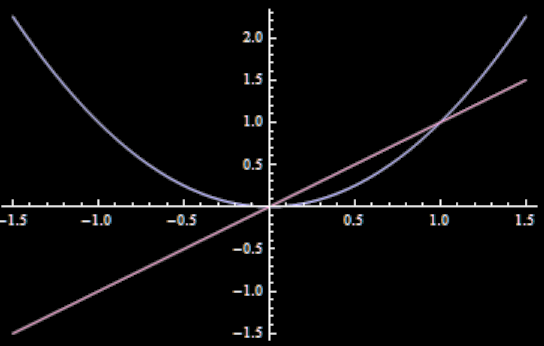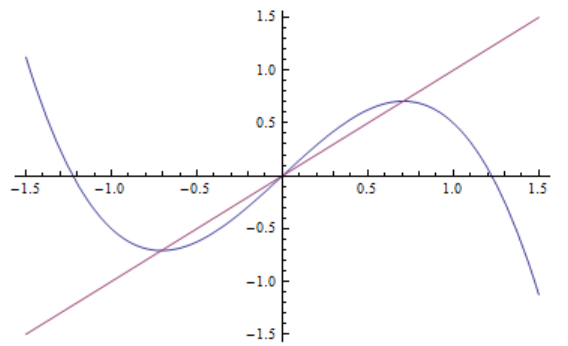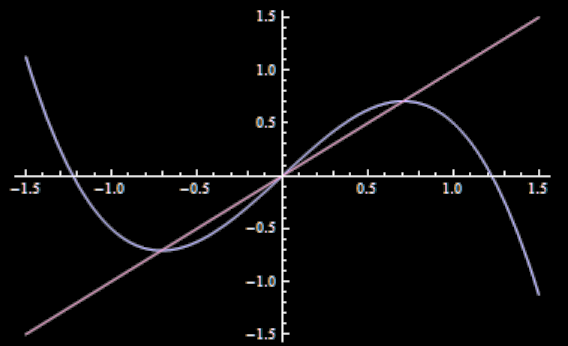# Standard ML Oracles vs Counterfactual ones

EDIT: This post has been superceeded by all these four posts.

A few weeks ago, I had a conversation with Paul Christiano about my counterfactual Oracle design.

We didn’t get as far as criticising the method. Paul’s question is far more fundamental—was my design even necessary?

# Forwards or backwards looking

The counterfactual oracle design was setup to reward the oracle for correctly guessing the ultimate value of a random variable (an outcome). This guess was conditional on humans not actually getting to see the guess.

If we ignore the counterfactual aspect for the moment, assume that the oracle has seen a series of past data: pairs . The are the background data the Oracle uses to estimate the values of the target variables .

Then my design is a forward-looking oracle. If we use a quadratic loss function, at turn , after seeing , it seeks to output , defined as:

• ,

where is the previous data (all including , all previous up to ).

Paul instead recommended using a more traditional machine-learning approach, where you attempt to fit a function that explains the past data, getting something like this:

• ,

where the are regularising terms that prevent overfitting. The oracle will then output . Call such oracle a backwards-facing oracle (BFO), because it only cares about the past.

Then the key facts about the BFO are:

• The BFO can generally cope with humans observing and modifying our behaviour because of it (ie does not need a counterfactual approach).

• The BFO is mildly manipulative in its outputs, though not generally in the way we fear an AI being.

• The BFO will not generally be manipulative in a way that minimises its cost function.

• However, the BFO may come to believe spurious correlations.

• Because of this, the BFO may converge more slowly—or not at all—where another oracle design could easily deduce the correct formulas.

# Observer perturbation effects

What happens if we observe the - and if this observation changes our behaviour so that itself is modified?

## Assume the oracle knows our plans and its actions

First of all, note that unless we set up the system very carefully, the oracle will “know” whether we are planning to observe or not. The counterfactual oracle was deliberately designed that observing is dependent on a purely stochastic process that is under the control of neither the human nor the oracle.

But in general, the Oracle will be able to deduce whether we expect to see the ; it can glean this information from the or from observing patterns in the past and . For the rest of this post, I’ll focus on the situation where we do indeed observe the ; if we don’t, then the BFO and the counterfactual oracle have similar behaviours to each other.

I’ll similarly assume that the BFO knows its own past outputs—the . It is possible for us to carefully setup the system so that it doesn’t track these, but I’ll assume that it has access to this information—either implicitly, through whatever process updates it’s estimate of the function , or explicitly, by deducing from and .

## Example

Assume for the moment that the are null and irrelevant, and that each is computed independently from all the others, but with identical distribution.

Ignoring the regularising terms in the cost function above, the best for the BFO is simply a constant, equal to the mean of the previous : .

If we observe (which, in this example, we assume we always do), then the value of is given by , where is a function and is a random variable with mean zero for all . If is continuous (and the possible value of are in a bounded interval), there will be fixed points where . We’ll expect the behaviour of the BFO to eventually converge to one of these fixed points. Note that, in the limit, if the BFO converges to the fixed point , then the cost function is equal to the expectation of .

For example, assume and - the relationship between observed and actual is deterministic. This has two fixed points: one at and one at .The point at is an attractive fixed point, but the point at is unstable. So unless all the data the BFO starts with has , the BFO will either fail to converge, or converge to .

We can add a non-trivial and this result still holds (indeed, a non-trivial just makes the point more unstable). What this means is that, even if the results in a lower cost function—ie - the BFO will still not converge to it, converging to the higher cost point .

## Multiple attractive fixed points

Let . This has three fixed points, at and . These last two points are attractive fixed points.If , then the BFO will converge to either or , depending on the initial data it starts with. If we add a non-trivial , we get a slight tendency to converge towards the fixed point with lower , everything else being equal. That’s because a large means that the next point sampled is likely to fall outside the “basin of attraction” of .

But everything else is not generally equal. The initial data strongly skews the process. The value of the derivative of around the fixed points (if it exists) affects the convergence process. The value of close to the fixed point (rather than at the fixed point) can have a large impact. Luck will play a role as well, if is non-trivial.

Thus, though there is a tendency to converge to fixed points with lower cost functions, this tendency is very weak. The BFO is not a cost function minimiser.

## Manipulation?

It is technically a manipulative act to output a such that we will change our value of in consequence.

But it’s not a manipulative act in most of the ways we fear. For example, if was the encoding for a program which, if run, would set , along with a convincing message for running that program, then is technically a fixed point of . However, it is not a fixed point that the BFO is likely to find, because would not be such an encoding for almost all , so the basin of attraction for this is tiny (basically only those sufficiently small to preserve all the digits of ). Thus the BFO is very unlikely to stumble upon it.

So this design does succeed in overcoming many of the problems with oracles: it will not try to manipulate us to obsessively converge on the point with lowest cost function.

# Problems with causality

So, what’s wrong with the BFO? I’ve written this much, pointing out its properties without endorsing it, so there must be something wrong.

Actually, I think it works very well for solving some problems, and, for those, is superior to my oracle designs. But it has certain limitations.

First of all, there’s the question of what happens if has no fixed points (for example, if is not continuous in various annoying ways) or no attractive fixed points (for example, if ). Then the BFO may not settle down in any stable way, as it continues to try and find the fixed point of a function without one.

But this problem is just a side effect of the more central point, which is that the BFO has a fundamentally wrong “understanding” of the causality of the situation (this is, of course, an informal phrasing—the BFO has no true “understanding” of the situation, in the ways we mean).

## Spurious beliefs

Let us go back to the and situation. Assume the is not empty, but is not relevant—for example, it could just be the time of day. Suppose the BFO has made three pairs of observations:

• , , .

If the BFO treated the as irrelevant (which they indeed are), its guess would be , and it would then eventually converge towards the fixed point .

But the BFO could also conclude that the parity of the time is relevant, and that it should output during even hours, and during odd hours.

If it does so, it will find confirmation for its theory. As it outputs , during even hours, it will observe that that guess was entirely correct, and similarly for odd hours.

In situations where has multiple attractive fixed points, and the BFO has a rich and varied amount of previous data for any reason, the BFO will find, and confirm, a spurious causality for explaning these different fixed points.

## False models

The problem is that the BFO’s greatest strength has become its weakness: it is trying to explain, through function fitting, why the seems to have different behaviours. We know that it is because of the , the output of the BFO itself; but the BFO cannot take this fact into account. Its function fitting is trying to implicitly take into account the output of its own calculations without explicitly doing so; no wonder that it ends up in Löbian situations.

This might result in the BFO being unable to converge to anything, in situations where the -minimising oracle could.

For example, we could imagine an environment where the and the are very varied, but the underlying causal structure is quite simple. An oracle that tracked as an input to the environment could deduce this causal structure quite easily.

But the BFO might struggle, as it tries to fit a function where it can’t explicitly take a key fact into account. Because the and are so varied, it should stumble upon many different spurious beliefs, making the job of fitting a single function to all the data extremely difficult. It would be interesting to explore the extent to which this might become a problem.

# In conclusion

The BFO/​traditional machine learning approach held up better than I supposed, and I’m thankful to Paul for bringing it to my attention. It has interesting advantages and drawbacks, and could be a useful oracle design in many situations.

• Unrelated to the effects of the outputs, I think the BFO approach might have the following limitation (this is inspired by your comment on a setup I once described):

It might be the case that in turn , when the programmers try to use the BFO to get answers to some hard questions, the “training distribution” at that point would be “easier” (or otherwise different) than the “test distribution” in some important sense, such that the BFO won’t provide useful answers. For example, perhaps a BFO couldn’t be used, soon after development, to answer: “If we give \$10,000,000 to a research lab X for their project Y, will the first FDA-approved cure for Alzheimer’s be approved at least twice sooner?”.

But even if that’s the case, a BFO might be sufficiently useful, even if it could always answer only questions that are not very different than the previous ones. A gradual process of answering harder and harder questions might provide sufficient value sufficiently quickly.

• The BFO can generally cope with humans observing and modifying our behaviour because of it (ie does not need a counterfactual approach).

Stuart, what’s your current opinion on backward-facing oracles? I ask because it seems like in a later post you changed your mind and went back to thinking that a counterfactual approach is needed to avoid manipulation after all. Is that right?

However, it is not a fixed point that the BFO is likely to find, because would not be such an encoding for almost all , so the basin of attraction for this is tiny (basically only those sufficiently small to preserve all the digits of ). Thus the BFO is very unlikely to stumble upon it.

This seems to constitute a form of overfitting or failure to generalize, that is likely to be removed by future advances in ML or AI in general (if there aren’t already proposed regularizers that can do so). (A more capable AI wouldn’t need to have actually “sumbled upon” the basin of attraction of this fixed point in its past data in order to extrapolate that it exists.) If you stick to using the “standard ML” BFO in order to be safe from such manipulation, wouldn’t you lose out in competitiveness against other AIs due to this kind of overfitting /​ failure to generalize?

• Stuart, what’s your current opinion on backward-facing oracles? I ask because it seems like in a later post you changed your mind and went back to thinking that a counterfactual approach is needed to avoid manipulation after all. Is that right?

Basically, yes. I’m no longer sure the terminology and concept of BFO is that useful, and I think all self-confirming oracles have problems. I also believe that these problems need not be cleanly of a “manipulation” or “non-manipulation” type.

I also believe there are smoother ways to reach “manipulation”, so my point starting “However, it is not a fixed point that the BFO is likely to find...” is wrong.

I’ll add a comment at the beginning of the post, clarifying this post is no longer my current best model.

• It’s not clear to me why the BFO would converge to a fixed point of . If we’ve solved the problem of embedded agency and the AI system knows that can depend on its prediction , then it would tend to find a fixed point, but it could also do the sort of counterfactual reasoning you say it can’t do. If we haven’t solved embedded agency, then it seems like the function that best explains the data is to posit the existence of some other classifier that works the same way that the AI did in past timesteps, and that . Intuitively, this is saying that the past data is explained by a hypothetical other classifier that worked the same way as the AI used to, and now the AI thinks one level higher than that. This probably does converge to a fixed point eventually, but at any given timestep the best hypothesis would be something that is a finite number of applications of and .

The BFO can generally cope with humans observing

Should this be ?